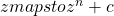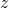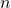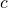fract variations

# fract variations

## Description

Escape-time fractals are in a way the opposite of flame fractals. Both use the idea of an orbit: the sequence of points generated by repeatedly iterating fractal formulas. But while flame fractals plot the actual orbit points to create the flame, escape-time fractals use the orbit of a point to determine how to color it, commonly by determining how long it takes the orbit to cross an imaginary circle (or to “escape”; hence the name “escape-time”). The two methods usually generate very different fractals.

Deep exploration of escape-time fractals is best done with software designed for that purpose. These programs usually support high precision calculations (required for deep zooms), lots of formulas, and alternate coloring methods. But the fract variations in JWildfire provide a way to include some escape-time fractals in flames. These are all “blur” variations that, like blur itself, ignore the input points and just output a random point in the given escape-time fractal. Like other blur variations, they can be part of a flame that repeats and distorts them, but are not themselves affected by other transforms.

All of the fract variations work in the same way and have mostly the same parameters. We’ll start with fract_mandelbrot_wf to understand how the parameters work, then examine the others to see the differences. For our starting point, we create a new flame, add a transform, and change the variation to fract_mandelbrot_wf. We’ll use the built-in gradient named Beautiful, and to make the colors pop better we change the Speed on the transform Color tab to -1 and change the Brightness on the global Coloring tab to 150. Here is the result, an image of the iconic Mandelbrot set:

Name: fract_dragon_wf
Type: 3D blur
Author: Andreas Maschke

Name: fract_julia_wf
Type: 3D blur
Author: Andreas Maschke

Name: fract_mandelbrot_wf
Type: 3D blur
Author: Andreas Maschke

Name: fract_meteors_wf
Type: 3D blur
Author: Andreas Maschke

Name: fract_pearls_wf
Type: 3D blur
Author: Andreas Maschke

Name: fract_salamander_wf
Type: 3D blur
Author: Andreas Maschke

The most critical parameter is the sixth one: buddhabrot_mode. The default is 0, which is normal escape-time mode. Setting it to 1 changes it to Buddhabrot mode, which is very different; we’ll come back to that after we’ve explored escape-time mode.

The xmin, xmax, ymin, and ymax parameters define a rectangle that bounds the escape-time fractal. Each time the fract variation is invoked, it picks a random point within this rectangle and computes its orbit to determine the escape time, which is used for coloring. It then adds the offsetx and offsety parameters and multiplies by the scale parameter and the variation amount to get the final (x,y) position.

As an example of how these parameters might be used, look closely at the Mandelbrot image above. At the top of the main cardoid (the large somewhat heart-shape) is a smaller bulb: a circle with smaller circles sticking out. Going up from that is a line that branches into two. Going up the left branch, near the end, is a small black dot. That dot is actually a tiny replica of the Mandelbrot set, often called a “minibrot”. Let’s zoom in on it. Set xmin to -0.2, xmax to -0.12, ymin to -1.08, ymax to -1.0, offsetx to 0.16 (the average of xmin and xmax, negated), offsety to 1.04 (similar calculation), and scale to 150. We now have a nice view of the minibrot!There are lots of minibrots–an infinite number. Near the top we can see a small but clear minibrot. Near the bottom right we see a sort of star shaped blob in the middle of a cross. That’s another minibrot. Here’s what it looks like when we zoom into it:It sort of looks like a minibrot, but isn’t well defined. To fix that, we need to increase the max_iter parameter. Here we triple it, to 300.Max_iter puts a limit on the size of orbits. If the orbit hasn’t escaped when max_iter iterations have been completed, it stops anyway. We need the limit because the orbits of points inside the minibrots will go on forever without escaping and we want our algorithm to terminate! Higher values for max_iter provide a more faithful image of the fractal, but it also increases the render time, so there is a tradeoff. A good rule of thumb is to leave it at 100 unless there is a need to increase it.

Hunting for minibrots is great fun! But JWIldfire isn’t really suited for it. Programs designed for rendering the Mandelbrot set make the job a lot easier. Besides better navigation features, they don’t produce the color shift seen here when max_iter is changed and they have better coloring options, such as smoothing algorithms that eliminate the banding seen here. Most also support higher precision which is required for deep zooms. (We’ve not gone not very deep, the scale is only 600 times the original.) So we won’t pursue it further.

To understand how the fract variations color fractals, let’s first review how JWildfire coloring works in general. It is based on a gradient and an index to the colors it contains. The index is a number between 0 and 1 (the left and right ends of the gradient). When a transform is selected, the current index is moved toward the transform’s Color setting according to the Speed setting. With Speed 0, the new index is halfway between the current value and the Color setting. With higher speed values, it moves less than halfway; at Speed 1 it doesn’t change. With lower speed values, it moves more than halfway; at Speed -1 the index is set to the Color setting.

If the direct_color parameter is set to 0, no additional change is made to the color index. The fract variation behaves just like other blur variations that don’t use direct color, taking a single color that is often a mixture of different gradient colors. The default and more typical use is to set direct_color to 1, which enables escape-time based coloring. This is done by dividing the escape time for a given point by the max_iter value, and adding that to the current color, adjusting the result so it is between 0 and 1.

For the examples above, we set the Color to 0 and the Speed to -1, ensuring that the color index given to the fract variation is always 0, and the resulting color for a point is determined solely by its escape time. Leaving Speed at -1 and changing Color has the same effect as shifting the gradient. Increasing Speed will start to overlap the colors, often softening the appearance of the banding that is typical of escape-time fractals. (The banding occurs because the escape time is always an integer, so doesn’t change continuously from one value to the next.) Of course, adding other transforms will also impact the colors generated by the fract variations.

The black areas in the images we have seen do not come from the gradient, but are actually “holes” where the black background shows through. These holes are created by “clipping” the fractal, and are controlled by the clip_iter_min and clip_iter_max parameters, which affect clipping on the outside and inside of the fractal, respectively. Looking back at the first image above, the straight edges on the top, bottom, and right sides are created by the ymin, ymax, and xmax parameters. The curved edges are created by clipping; clip_iter_min is 3, which clips points with an escape time of 3 or less. Setting clip_iter_min to 1 will not clip on the outside, showing the entire rectangle; here it shows two more bands. Increasing clip_iter_min will clip more bands; in the right image below clip_iter_min is set to 6.Clip_iter_max clips the inside of the fractal. Setting it to 0 will prevent clipping; the inside will be filled with a solid color. Reducing it to -1 will clip all points whose orbit reached the maximum length (max_iter) without escaping. Reducing it further will clip bands lining the inside of the fractal, which are not usually visible. So in most cases, leave it at the default value -5 to clip the inside or set it to 0 to fill the inside.

The last clip parameter has a somewhat confusing name, max_clip_iter. As noted previously, the fract variations start by picking a random point within the boundary rectangle and computing its orbit. If it turns out that point is clipped by clip_iter_min or clip_iter_max, another random point is chosen and the process repeated. After this happens max_clip_iter times without success, the variation gives up. Sometimes this can cause artifacts like spurious dots in the flame. If this happens, try increasing max_clip_iter. But be careful; high values can slow down rendering to the point that JWildfire appears to hang.

The fractals computed by the fract variations are two dimensional, but the variations use the escape time to set z, adding height to the fractal. Points that are close to the inside will have a high escape time and be given more height. The following image shows the effect; it is the same as the first image above, but with the pitch increased to 50°.The height can be controlled with the scalez parameter, and is also affected by the scale parameter and the variation amount. There is also an offsetz parameter that shifts the entire result up or down, and a z_logscale parameter that, if set to 1, adds a log operation that can help make the rise less steep.

If scale is set to a large value, the z values will probably be far too large. This can be adjusted using scalez. For example, taking the zoomed-in image above where scale is 2400, we compensate by setting scalez to 0.005 and change the pitch to 50°:As we have already seen, the escape time is an integer which causes banding in the coloring of the 2D fractal. This also has an impact on the height: the z value for the points within a band will be the same, but jump to a different value when the color changes, leaving gaps. In most cases, the gaps are not apparent, but when the parameter z_fill is larger than 0 the gaps will be filled in. The value represents the density of the fill, with 1 being the maximum.

To see how this works, we contrive an example by viewing the Mandelbrot site edge on (pitch=90°), reducing max_iter to 50, and setting zscale to 20. On the left, with z_fill=0, the gaps between the layers can be seen. On the right, with z_fill=1, the gaps are filled in.Buddhabrot mode is very different from escape-time mode. It is sort of a cross between and escape-time and orbital fractal. If we take the first Mandelbrot example above, set buddhabrot_mode to 1, turn the camera 90° clockwise, and reduce the Gamma to 1.5, we get the following, which shows how this mode gets its name: it is reminiscent of a classical depiction of the Buddha.Buddhabrot mode works by randomly picking a point whose orbit escapes from the rectangle defined by the xmin, xmax, ymin, and ymax parameters. It then plots the orbit points up to max_iter (it doesn’t stop when it escapes). The process is then repeated with a new random point. The result is a kind of density plot highlighting the areas where the orbits spend most of their time.

The parameter buddhabrot_min_iter specifies the minimum iteration that is plotted, allowing the initial orbit points to be ignored. This can subtly change the appearance of the Buddhabrot fractal. Buddhabrot mode does not do clipping, does not set z, and always uses direct color; the parameters associated with these features are ignored.

Here are a few hints for using Buddhabrot mode:

• Low values for Gamma (on the Coloring tab) can help enhance the features of the Buddhabrot itself as well as reduce the haze around the outside. This was done for the image above.
• The value of max_iter can have a large effect on the result. Increasing it tends to bring out fine details, but muddles the colors. Decreasing it tends to make a more colorful image (with the right gradient), but also causes some distortion. But that distortion can be interesting; give it a try. Changing max_iter also affects the overall brightness, requiring changes to the Brightness setting.
• The bounding rectangle defined by xmin, xmax, ymin, and ymax do not clip the resulting Buddhabrot. However, they do affect it since it restricts where the initial points are taken. It’s best to turn off Buddhabrot mode when setting them to make sure the rectangle contains plenty of outside points. If most of the points are inside, the Buddhabrot computation will be inefficient and dim. (If all the points are inside, it will hang JWildfire!)
• The scale, offsetx, and offsety parameters work in Buddhabrot mode the same as in escape-time mode.

The following image was made by setting the bounding rectangle to the cusp on the right side of the Mandelbrot set and reducing max_iter to 40 to introduce some distortion. The scale and offsetx parameters were used to make the shape fit the image. The bright spot in the middle is from the background, which was set to white.We will now look at each of the individual fract variations, starting with fract_mandelbrot_wf since we have been using it. It has one additional parameter, power. Its formula is, whereis the complex number for the point (x,y) (which is not related to the 3D z value),is the value of the power parameter andis set to the starting pixel of the orbit. The default power of 2 produces the standard Mandelbrot set, as shown above. Increasing it changes the shape and makes it symmetric; the following image uses a power of 7. If power is increased, the values of xmin, xmax, ymin, ymax, and offsetx will need to be adjusted to fit the new fractal.Fract_julia_wf variation uses the same formula:. But in this case,is the same for all points, and specified by the xseed and yseed parameters (xseed is the real part and yseed the imaginary part of the complex number. Here are a the results of a few different xseed and yseed values. In the left image, xseed=0.3 and yseed=0.5. In the middle image, xseed=0.268 and yseed=0.0. In the right image, xseed=0.22 and yseed=-0.588.There are, of course, many other possibilities. But they can be divided into two types: Julia fractals with an inside (such as the left one) with points whose orbits do not escape, and Julia fractals with no inside (the other two), whose orbits always escape. We can tell whether a Julia fractal has an inside by determining whether the origin (0,0) escapes or not.

The default values for fract_julia_wf make an especially interesting fractal:There are a lot of black holes that seem to be inside points, but the origin, in the middle, is not black, so it shouldn’t have any. In fact, the holes are not really inside points; they only appear because max_iter cuts off the orbits of those points before they escape. Increasing max_iter will make the holes smaller.

Buddhabrot mode is not generally very interesting with fract_julia_wf. It produces results similar in form to escape-time mode, but they are overall very dim with very bright spots that are difficult to deal with. But the 3D effect of setting z according to the escape time can be interesting; here is a solid 3D render using only fract_julia_wf:Fract_dragon_wf is similar to fract_julia_wf, but uses a different formula so produces different results. In general, max_iter needs to be higher with fract_dragon_wf; it is set to 250 in the examples below. The xseed and yseed parameters are very sensitive to small changes. Click on Fine Edit (above the preview on the left) before dragging in the parameter field to give more control over the shape.Fract_salamander_wf uses yet another formula. Use it in the same way as the others. It produces square results if xmin, xmax, ymin, and ymax are set appropriately. Increase clip_iter_min to 1 to make the result a circle.Fract_meteors_wf works in the same way as fract_mandelbrot_wf, but uses a very different formula that tends to produce lots of circles of all sizes (the meteors?). There are no extra parameters; here is the basic fractal:Like fract_mandelbrot_wf, zooming in can produce interesting results, but requires increasing max_iter so the coloring changes.For 3D results, it is especially nice to set clip_iter_max to 0 so the inside is filled in, and set scalez to a negative value so the inside changes from a mesa to a lake. Note that the “shadows” here are actually holes showing the black background.This one also works well in Buddhabrot mode. The scale parameter needs to be reduced a bit, as does the gamma value.Fract_pearls_wf produces a fractal that can range in appearance from a string of pearls to a mesh of pearls to a mass of pearls, depending on the xseed and yseed parameters. By default, clip_iter_min is 1, so the entire square defined by xmin, xmax, ymin, and ymax is shown. Increase it to 2 and it becomes a circle. Increase it further, and the inside circles start disappearing.The orbits jump from circle to circle, so Buddhabrot mode is similar to escape-time mode, but fainter and with difficult bright spots; not very useful. The 3D results are nice: circles with different colors are at different heights. It works well with either positive or negative scalez.

The fract variations make interesting fractals when used alone, as seen in all of the above images. But the main reason for using them in JWildfire is to incorporate them into flames. Fract_pearls_wf and fract_salamander_wf are both squares with the default parameters, and circles when clip_iter_min is set to 2, which makes them particularly easy to substitute anywhere a square or circle blur is used. For example, here is a plastic style image (see the spherical variation information), but using fract_salamander_wf in place of blur.Since all of the fract variations produce a rectangle when we set clip_iter_min to 1, it is easy to tile them. To simplify the calculations, we set scale to 1 along with the variation amount, offsetx to the negative of the average of xmin and xmax, and offsety similarly. Then we add four variations with linear3D. In the Affine tab, on the first set O1 to xmax-xmin, the second set O1 to xmin-xmax, the third set O2 to ymax-ymin, and the last set O2 to ymin-ymax. Using fract_julia_wf and making adjustments to the Xaos and Color settings we get something like this:We can then turn the tiling into something more interesting. For example, we add a final with variation sin and scale it down by π/3 to line up the tiles. Then we take advantage of the 3D feature of the fract variation by increasing the Pitch and adding zscale to the final sin. (We could have set Preserve Z, but using zscale allows further adjustment if needed.) Finally, we add a second final with variation ovoid3d and adjust the z parameters of fract_julia_wf to get this:## Related Variations

There are two more fract variations: fract_formula_mand_wf and fract_formula_julia_wf. These are intended to be like fract_mandelbrot_wf and fract_julia_wf, but allowing the formula to be specified. However, there are bugs in their implementation in JWildfire 4.00 and earlier, so they don’t always produce good results at the present time. If you want to try them anyway, here are some hints:

• Most of the parameters are the same as the other fract variations described above. They have an additional parameter called formula to change the formula. To do so, click the gear icon to the right.
• The formula must be fully parenthesized, including a set or parentheses around the entire formula. For example, the default formula for fract_formula_mand_wf is “((z^n)+c)”. Valid operators are +, -, *, /, and ^. Valid letters are z (the previous orbit point), c (the starting point for fract_formula_mand_wf or the seed value for fract_formula_julia_wf), n (the power parameter), and i (the imaginary number). Z and c are complex numbers, but their real and imaginary parts can be accessed using x and y for z, and a and b for c. Positive constants can also be used (e.g., 3.14).
• In JWildfire version 4.00 and earlier, multiplication and division (* and /) do not work properly.
• An incorrectly formatted formula is likely to hang JWildfire. So be sure to save anything you need before playing with it.

There is also a variation named mandelbrot which is similar to fract_mandelbrot_wf. It has parameters iter, xmin, xmax, ymin, and ymax like the fract variations, but the other parameters are very different so it produces different results, and it has no direct color option.

## Parameters

Flame pack (flames used above)